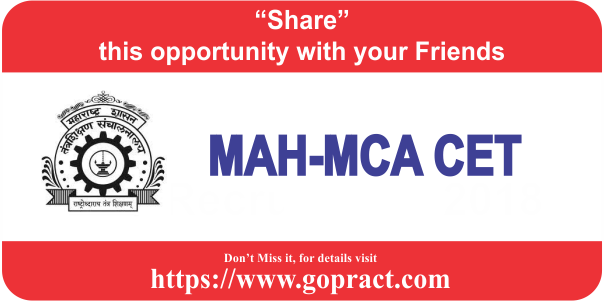# MAH MCA CET 2022

Share Now
The Competent Authority will be conducting the Online MAH- MCA-CET 2022 for admission to first year / direct second year (separate division & lateral entry) of three year full time post graduate degree course in Master of Computer Applications (MCA), for Academic Year 2022-23.### Important Dates

 Online registration for MAH-MBA/MMS CET 2022 17 Mar 2022 Closure of registration of application 11 May 2022 Final Exam 04 and 05 Aug 2022

### SCHEME OF EXAMINATION

The MAH-MCA CET consists of four question papers. With total of 200 marks.

SR.NOSubjectNo of QuestionsMark(s) per questionMaximum Marks Total Marks
1Mathematics & Statistics30260200
2Logical / Abstract Reasoning30260
3English comprehension and verbal ability20240
4Computer Concepts 20240

The test will comprise of multiple choice objective type questions with four options. Candidate have to choose 1 correct answer out of it

Mode of examination is Online with total tests duration of 90 minutes. The medium of test is English and there will not be any negative marking for MAH-MCA CET 2022

### Syllabus for Master of Computer Applications MAH-MCA-CET 2022 in detail

The Online CET would comprise 4 sections viz. Mathematics & Statistics, Logical / Abstract Reasoning, English comprehension and verbal ability and Computer Concepts of total 200 marks , with composite time of 90 minutes duration

.

#### Syllabus

1. Mathematics & Statistics: The questions will cover the following topics of high school mathematics (up to the 12th standard)
• Algebra : Fundamental operations in Algebra, Expansion, factorization, Quadratic equations, indices, logarithms, arithmetic, geometric and harmonic progressions, binomial theorem, permutations and combinations.
• Co-ordinate Geometry : Rectangular Cartesian co-ordinates, equations of a line, mid point, intersections etc.,equations of a circle, distance formulae, pair of straight lines, parabola, ellipse and hyperbola, simple geometric transformations such as translation, rotation, scaling.
• Differential Equations: Differential equations of first order and their solutions, linear differential equations with constant coefficients, homogeneous linear differential equations.
• Trigonometry: Simple identities, trigonometric equations, properties of triangles, solution of triangles, height and distance, inverse function.
• Probability and Statistics : Basic concepts of probability theory, Averages, Dependent and independent events,frequency distributions, and measures of dispersions, skewness and kurtosis, random variable and distribution functions, mathematical expectations, Binomial, Poisson, normal distributions, curve fitting, and principle of least squares, correlation and regression.
• Arithmetic: Ratios and proportions, problems on time-work, distance-speed, percentage, etc.
• Basic Set Theory and Functions: Set, relations and mappings.
• Mensuration: areas, triangles and quadrilaterals, area and circumference of circles, volumes and surface areas of simple solids such as cubes, spheres, cylinders and cones.
2. Logical / Abstract Reasoning: This shall include the questions to measure how quickly and logically you can think. This section will cover logical situations and questions based on the facts given in the passage. This test shall check the problem solving capability of the candidate.
3. English comprehension and verbal ability: Questions in this section will be designed to test the candidate’s general understanding of the English language. There will be questions on the topics such as Basic English grammar, vocabulary, comprehension, synonyms, antonyms, sentence correction, word & phrases, jumbled paragraphs.
4. Computer Concepts
• Computer Basics : Organization of a computer, Central Processing Unit (CPU), Structure of instructions in CPU, input / output devices, computer memory, memory organization, back-up devices.
• Data Representation : Representation of characters, integers, and fractions, binary and hexadecimal representations, Binary Arithmetic: Addition, subtraction, division, multiplication, signed arithmetic and two’s complement arithmetic, floating point representation of numbers, normalized floating point representation, Boolean algebra, truth tables, Venn diagrams.
• Computer Architecture: Basics of Digital Logic ,Block structure of computers, communication between processor and I / O devices, interrupts.
• Computer Language: Fundamentals of Data & File Structures and high level language, Computer Programming in C, C++, advanced concepts in programming.
• Operating System basics

### Educational Qualification

1. The candidate must be a Citizen of India.
2. The candidate should be an Indian National;
3. Passed minimum three year duration Bachelor’s Degree awarded by University recognised by University Grants Commission or Association of Indian Universities in any discipline with at least 50% marks in aggregate or equivalent (at least 45% in case of candidates of backward class categories and Persons with Disability belonging to Maharashtra State only);
4. Studied Mathematics as one of the subjects at (10+2) level or at Graduate level examination;
5. For Direct second year admissions, the candidate should have passed Bachelor Degree in Computer Applications or Science (Information Technology or Computer Science);